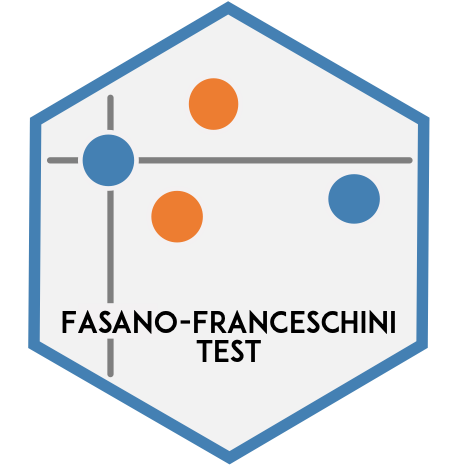# Fasano-Franceschini Test

The `fasano.franceschini.test` package is an R implementation of the multidimensional Kolmogorov-Smirnov two-sample test as defined by Fasano and Franceschini (1987).

``````Fasano, G. & Franceschini, A. (1987). A multidimensional version of the
Kolmogorov-Smirnov test. Monthly Notices of the Royal Astronomical Society,
225:155-170. doi: 10.1093/mnras/225.1.155.``````

## Installation

You can install the released version of the `fasano.franceschini.test` package from CRAN with:

``install.packages("fasano.franceschini.test")``

The development version of the `fasano.franceschini.test` package can be installed from Github with:

``````# install.packages("devtools")
devtools::install_github("braunlab-nu/fasano.franceschini.test")``````

## Examples

#### Underlying distributions are the same

``````library(fasano.franceschini.test)

# set seed for reproducibility
set.seed(0)

# create 2D samples from the same underlying distribution
S1 <- data.frame(x = rnorm(n = 50, mean = 0, sd = 1),
y = rnorm(n = 50, mean = 0, sd = 3))
S2 <- data.frame(x = rnorm(n = 100, mean = 0, sd = 1),
y = rnorm(n = 100, mean = 0, sd = 3))

fasano.franceschini.test(S1, S2, seed = 0)
#>
#>  Fasano-Franceschini Test
#>
#> data:  S1 and S2
#> D = 1700, p-value = 0.6333``````

#### Underlying distributions are different

``````# set seed for reproducibility
set.seed(1)

# create 3D samples from different underlying distributions
S1 <- cbind(rgamma(n = 43, shape = 2),
rpois(n = 43, lambda = 5),
rpois(n = 43, lambda = 3.5))
S2 <- cbind(rgamma(n = 72, shape = 2),
rpois(n = 72, lambda = 5),
rpois(n = 72, lambda = 5))

fasano.franceschini.test(S1, S2, seed = 1)
#>
#>  Fasano-Franceschini Test
#>
#> data:  S1 and S2
#> D = 2022, p-value = 0.01977``````